Publicité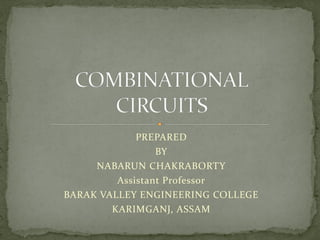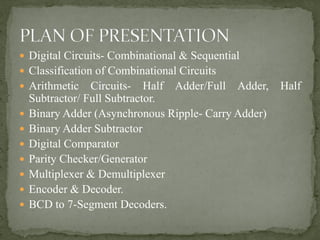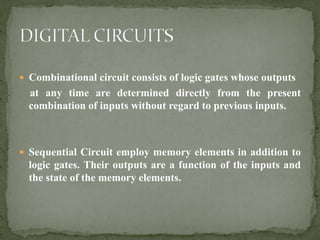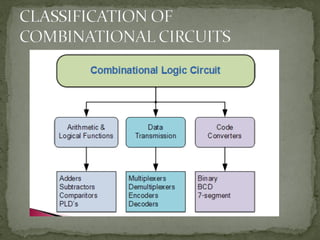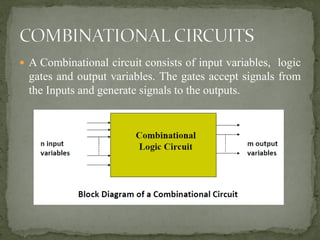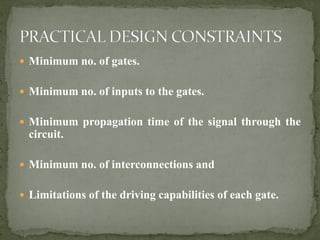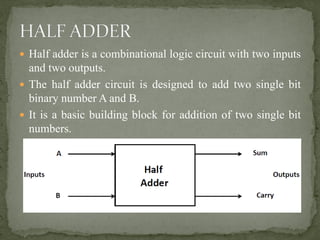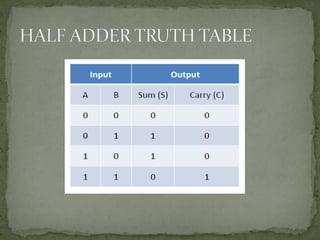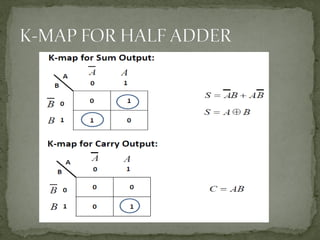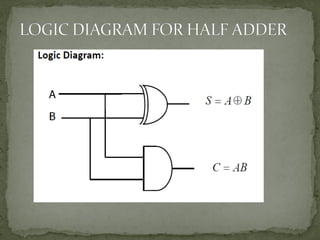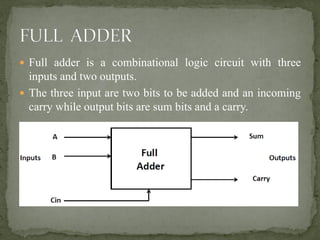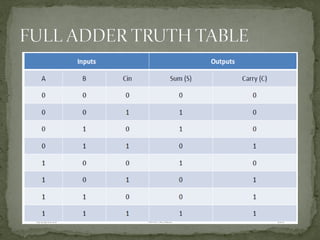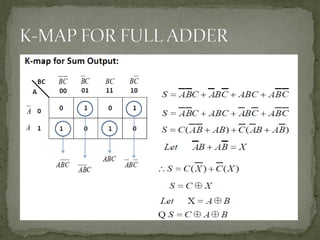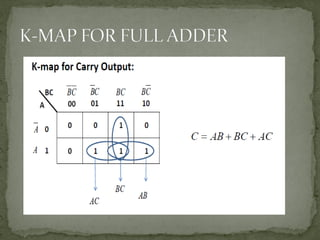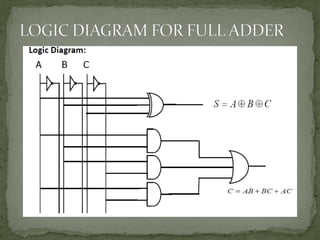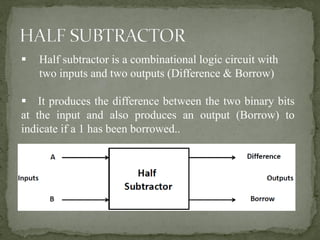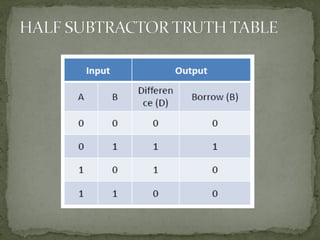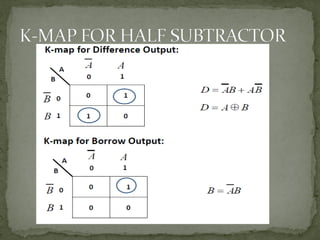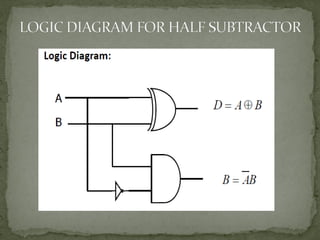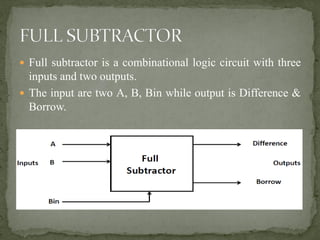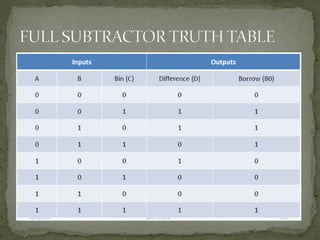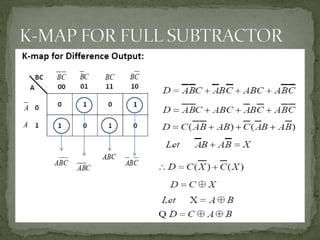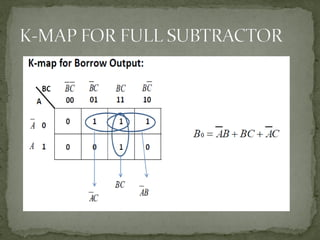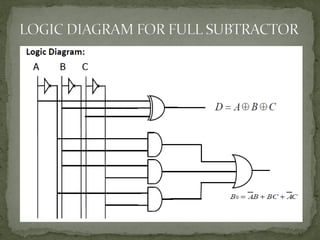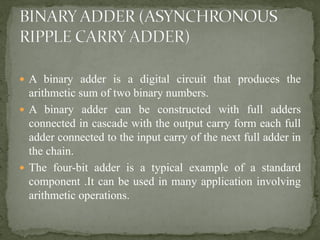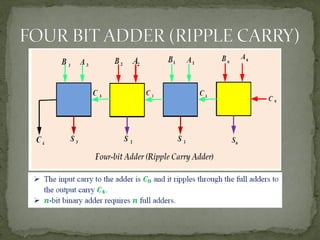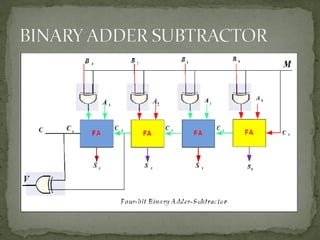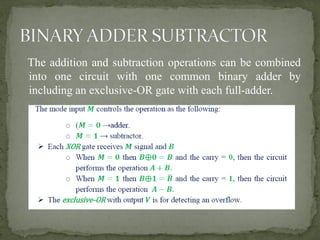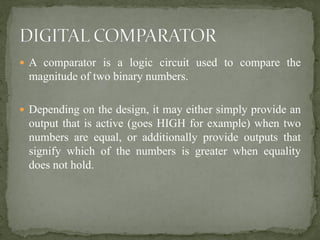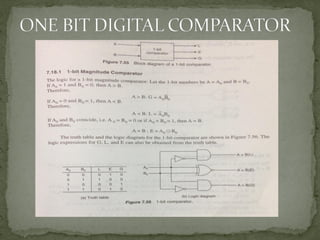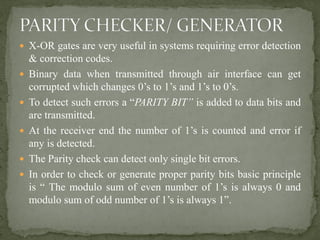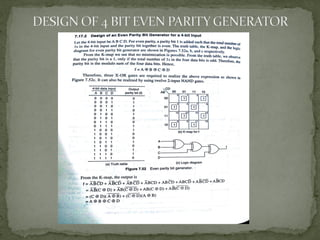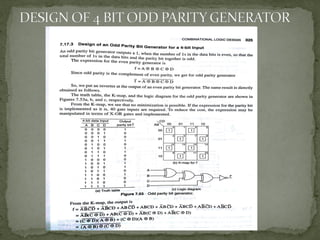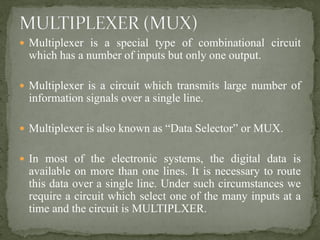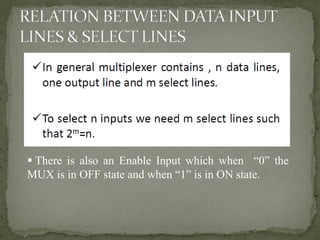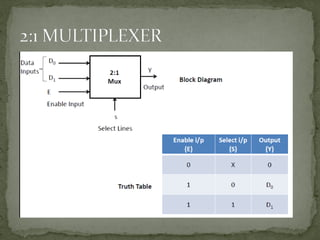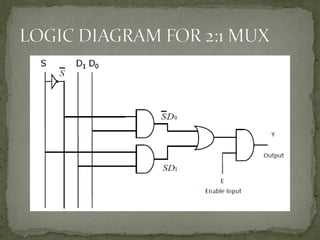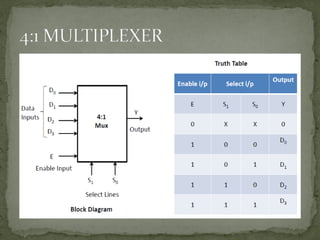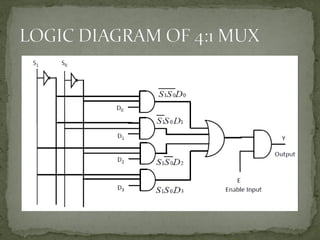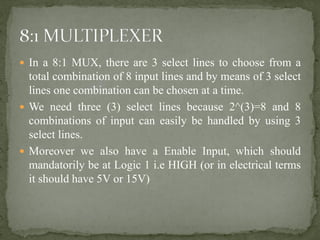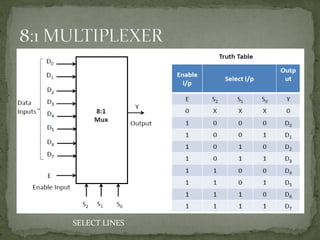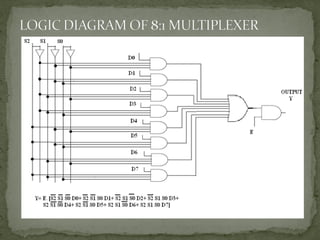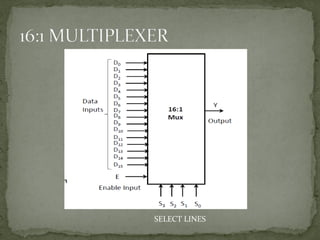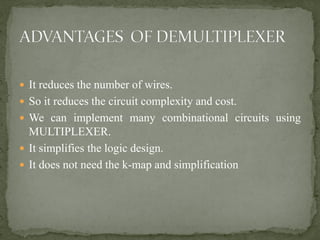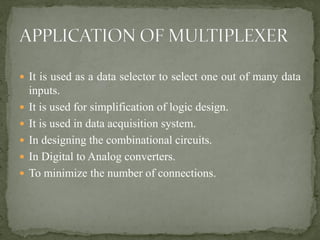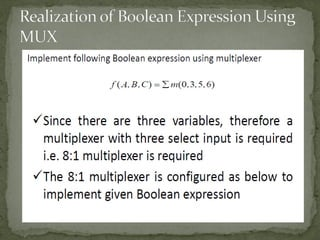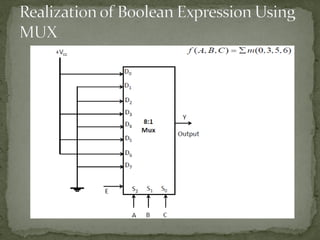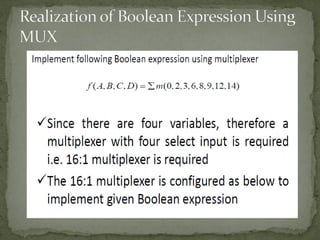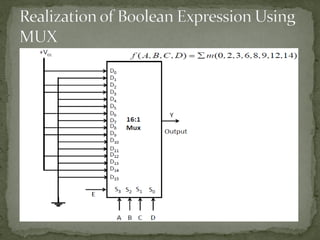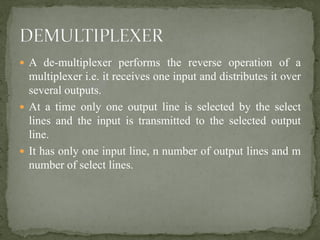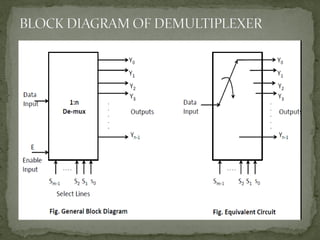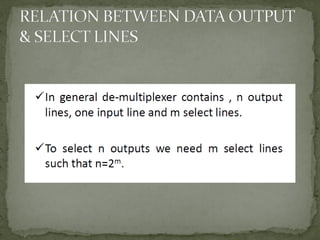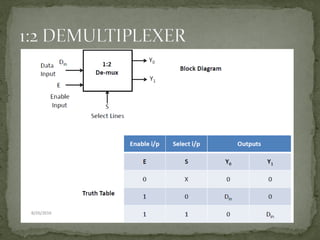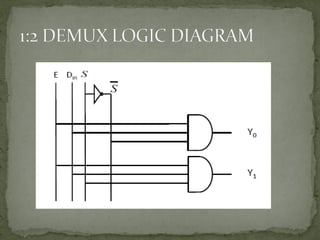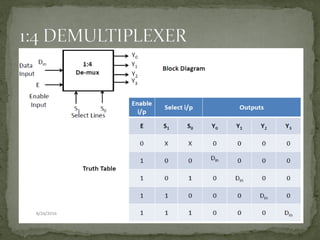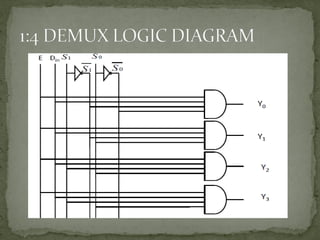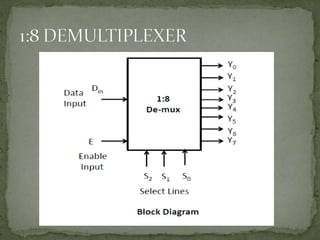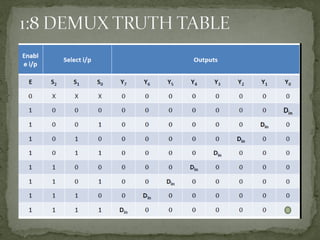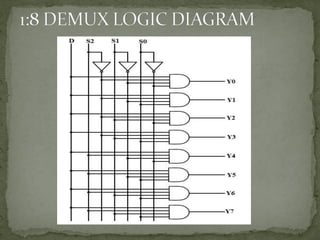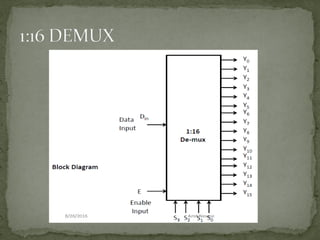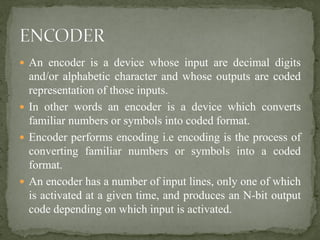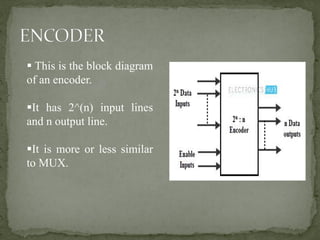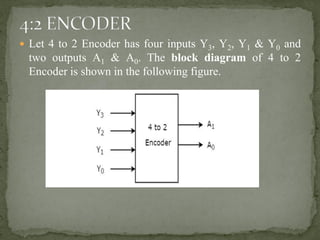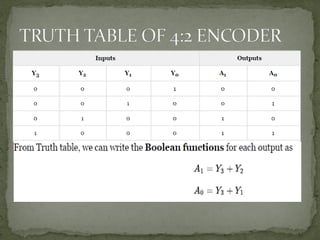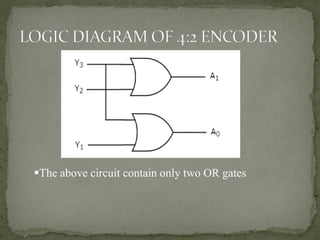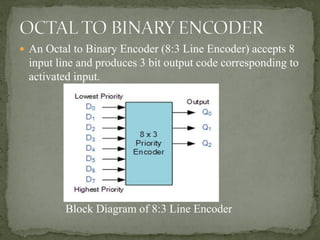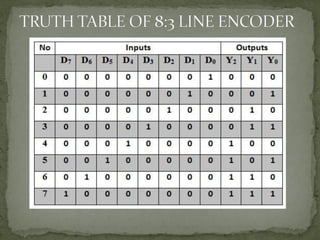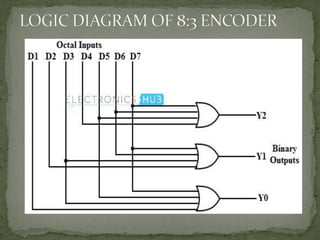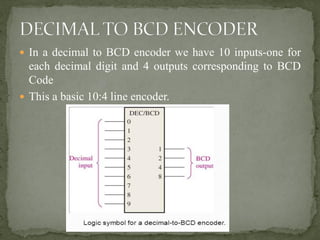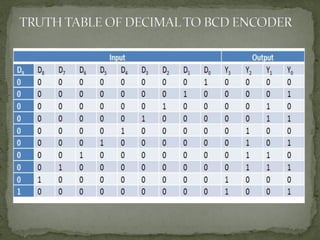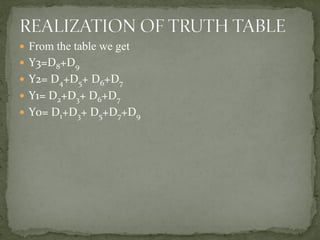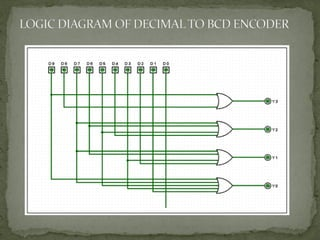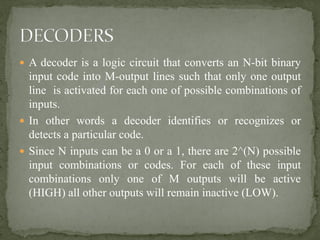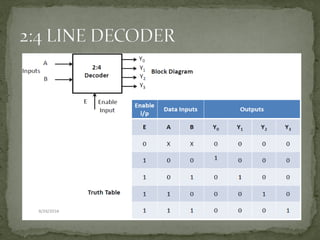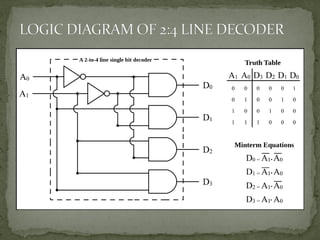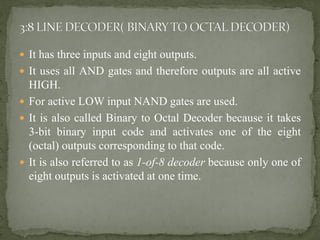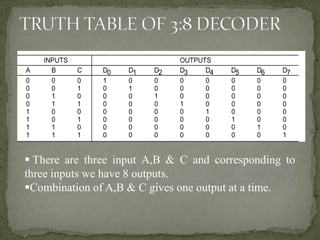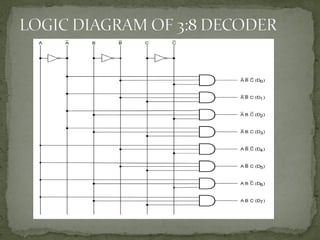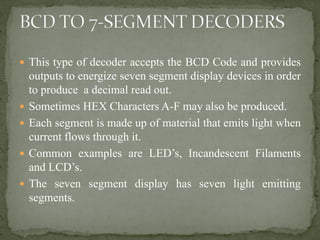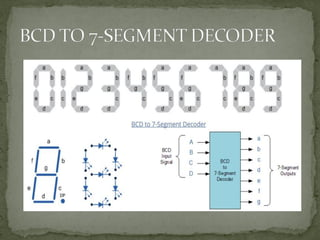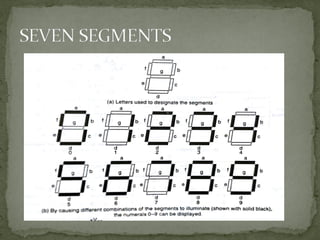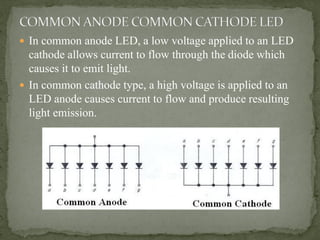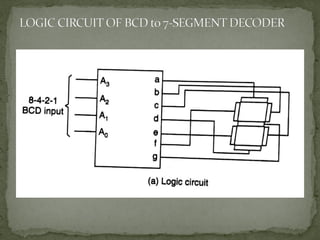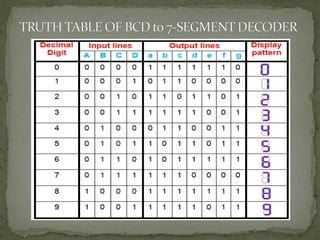1 sur 86
Publicité

### Combinational circuits

1. PREPARED BY NABARUN CHAKRABORTY Assistant Professor BARAK VALLEY ENGINEERING COLLEGE KARIMGANJ, ASSAM
2.  Digital Circuits- Combinational & Sequential  Classification of Combinational Circuits  Arithmetic Circuits- Half Adder/Full Adder, Half Subtractor/ Full Subtractor.  Binary Adder (Asynchronous Ripple- Carry Adder)  Binary Adder Subtractor  Digital Comparator  Parity Checker/Generator  Multiplexer & Demultiplexer  Encoder & Decoder.  BCD to 7-Segment Decoders.
3.  Combinational circuit consists of logic gates whose outputs at any time are determined directly from the present combination of inputs without regard to previous inputs.  Sequential Circuit employ memory elements in addition to logic gates. Their outputs are a function of the inputs and the state of the memory elements.
4.  A Combinational circuit consists of input variables, logic gates and output variables. The gates accept signals from the Inputs and generate signals to the outputs.
5.  Minimum no. of gates.  Minimum no. of inputs to the gates.  Minimum propagation time of the signal through the circuit.  Minimum no. of interconnections and  Limitations of the driving capabilities of each gate.
6.  Half adder is a combinational logic circuit with two inputs and two outputs.  The half adder circuit is designed to add two single bit binary number A and B.  It is a basic building block for addition of two single bit numbers.
7.  Full adder is a combinational logic circuit with three inputs and two outputs.  The three input are two bits to be added and an incoming carry while output bits are sum bits and a carry.
8. ▪ Half subtractor is a combinational logic circuit with two inputs and two outputs (Difference & Borrow) ▪ It produces the difference between the two binary bits at the input and also produces an output (Borrow) to indicate if a 1 has been borrowed..
9.  Full subtractor is a combinational logic circuit with three inputs and two outputs.  The input are two A, B, Bin while output is Difference & Borrow.
10.  A binary adder is a digital circuit that produces the arithmetic sum of two binary numbers.  A binary adder can be constructed with full adders connected in cascade with the output carry form each full adder connected to the input carry of the next full adder in the chain.  The four-bit adder is a typical example of a standard component .It can be used in many application involving arithmetic operations.
11. The addition and subtraction operations can be combined into one circuit with one common binary adder by including an exclusive-OR gate with each full-adder.
12.  A comparator is a logic circuit used to compare the magnitude of two binary numbers.  Depending on the design, it may either simply provide an output that is active (goes HIGH for example) when two numbers are equal, or additionally provide outputs that signify which of the numbers is greater when equality does not hold.
13.  X-OR gates are very useful in systems requiring error detection & correction codes.  Binary data when transmitted through air interface can get corrupted which changes 0’s to 1’s and 1’s to 0’s.  To detect such errors a “PARITY BIT” is added to data bits and are transmitted.  At the receiver end the number of 1’s is counted and error if any is detected.  The Parity check can detect only single bit errors.  In order to check or generate proper parity bits basic principle is “ The modulo sum of even number of 1’s is always 0 and modulo sum of odd number of 1’s is always 1”.
14.  Multiplexer is a special type of combinational circuit which has a number of inputs but only one output.  Multiplexer is a circuit which transmits large number of information signals over a single line.  Multiplexer is also known as “Data Selector” or MUX.  In most of the electronic systems, the digital data is available on more than one lines. It is necessary to route this data over a single line. Under such circumstances we require a circuit which select one of the many inputs at a time and the circuit is MULTIPLXER.
15. ▪ There is also an Enable Input which when “0” the MUX is in OFF state and when “1” is in ON state.
16.  In a 8:1 MUX, there are 3 select lines to choose from a total combination of 8 input lines and by means of 3 select lines one combination can be chosen at a time.  We need three (3) select lines because 2^(3)=8 and 8 combinations of input can easily be handled by using 3 select lines.  Moreover we also have a Enable Input, which should mandatorily be at Logic 1 i.e HIGH (or in electrical terms it should have 5V or 15V)
17. SELECT LINES
18. SELECT LINES
19.  It reduces the number of wires.  So it reduces the circuit complexity and cost.  We can implement many combinational circuits using MULTIPLEXER.  It simplifies the logic design.  It does not need the k-map and simplification
20.  It is used as a data selector to select one out of many data inputs.  It is used for simplification of logic design.  It is used in data acquisition system.  In designing the combinational circuits.  In Digital to Analog converters.  To minimize the number of connections.
21.  A de-multiplexer performs the reverse operation of a multiplexer i.e. it receives one input and distributes it over several outputs.  At a time only one output line is selected by the select lines and the input is transmitted to the selected output line.  It has only one input line, n number of output lines and m number of select lines.
22.  An encoder is a device whose input are decimal digits and/or alphabetic character and whose outputs are coded representation of those inputs.  In other words an encoder is a device which converts familiar numbers or symbols into coded format.  Encoder performs encoding i.e encoding is the process of converting familiar numbers or symbols into a coded format.  An encoder has a number of input lines, only one of which is activated at a given time, and produces an N-bit output code depending on which input is activated.
23. ▪ This is the block diagram of an encoder. ▪It has 2^(n) input lines and n output line. ▪It is more or less similar to MUX.
24.  Let 4 to 2 Encoder has four inputs Y3, Y2, Y1 & Y0 and two outputs A1 & A0. The block diagram of 4 to 2 Encoder is shown in the following figure.
25. ▪The above circuit contain only two OR gates
26.  An Octal to Binary Encoder (8:3 Line Encoder) accepts 8 input line and produces 3 bit output code corresponding to activated input. Block Diagram of 8:3 Line Encoder
27.  In a decimal to BCD encoder we have 10 inputs-one for each decimal digit and 4 outputs corresponding to BCD Code  This a basic 10:4 line encoder.
28.  From the table we get  Y3=D8+D9  Y2= D4+D5+ D6+D7  Y1= D2+D3+ D6+D7  Y0= D1+D3+ D5+D7+D9
29.  A decoder is a logic circuit that converts an N-bit binary input code into M-output lines such that only one output line is activated for each one of possible combinations of inputs.  In other words a decoder identifies or recognizes or detects a particular code.  Since N inputs can be a 0 or a 1, there are 2^(N) possible input combinations or codes. For each of these input combinations only one of M outputs will be active (HIGH) all other outputs will remain inactive (LOW).
30.  It has three inputs and eight outputs.  It uses all AND gates and therefore outputs are all active HIGH.  For active LOW input NAND gates are used.  It is also called Binary to Octal Decoder because it takes 3-bit binary input code and activates one of the eight (octal) outputs corresponding to that code.  It is also referred to as 1-of-8 decoder because only one of eight outputs is activated at one time.
31. ▪ There are three input A,B & C and corresponding to three inputs we have 8 outputs. ▪Combination of A,B & C gives one output at a time.
32.  This type of decoder accepts the BCD Code and provides outputs to energize seven segment display devices in order to produce a decimal read out.  Sometimes HEX Characters A-F may also be produced.  Each segment is made up of material that emits light when current flows through it.  Common examples are LED’s, Incandescent Filaments and LCD’s.  The seven segment display has seven light emitting segments.
33.  In common anode LED, a low voltage applied to an LED cathode allows current to flow through the diode which causes it to emit light.  In common cathode type, a high voltage is applied to an LED anode causes current to flow and produce resulting light emission.
Publicité